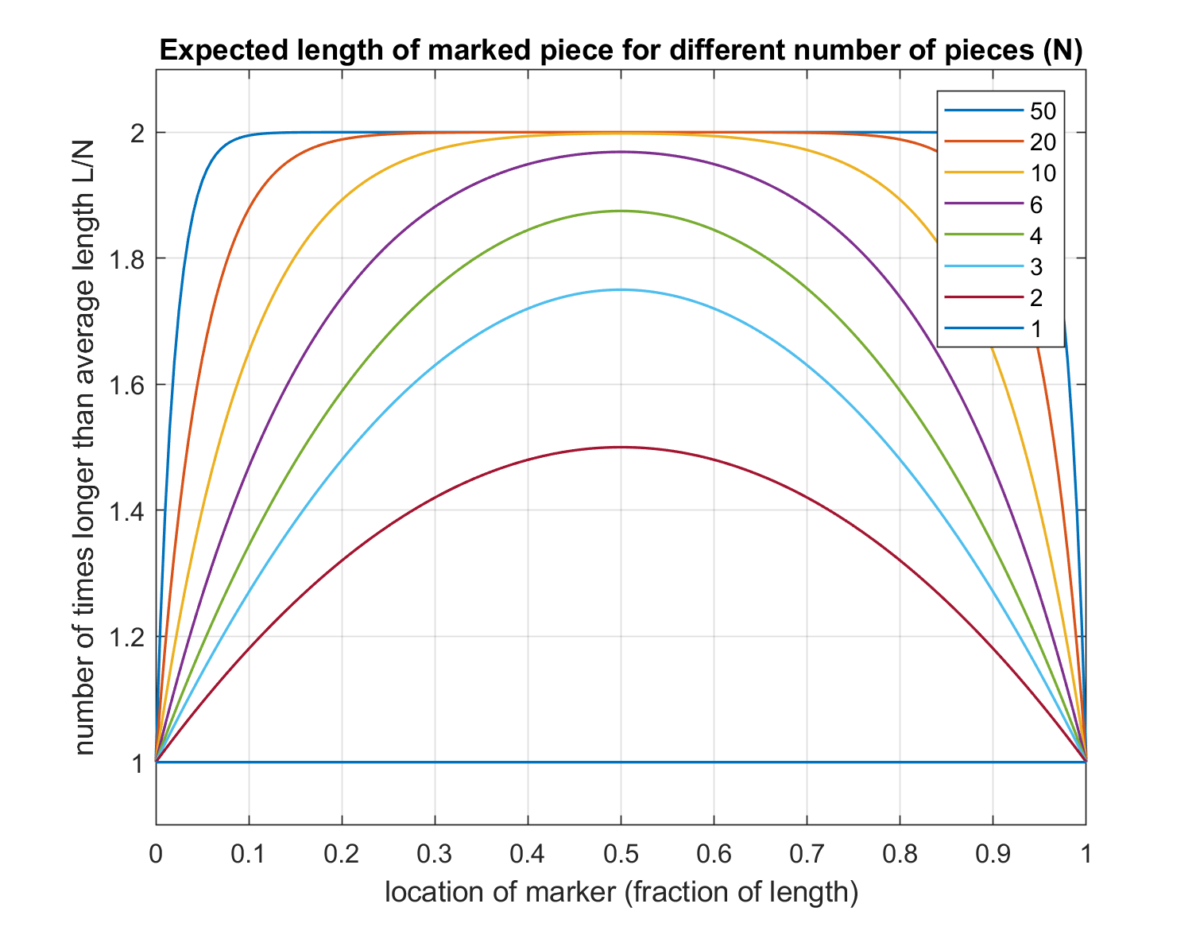# Cutting a ruler into piecesThis week’s Riddler Classic is a paradoxical question about cutting a ruler into smaller pieces.

Recently, there was an issue with the production of foot-long rulers. It seems that each ruler was accidentally sliced at three random points along the ruler, resulting in four pieces. Looking on the bright side, that means there are now four times as many rulers — they just happen to have different lengths. On average, how long are the pieces that contain the 6-inch mark?

With four cuts, each piece will be on average 3 inches long, but that can’t be the answer, can it?

Here is my solution:
[Show Solution]

## 6 thoughts on “Cutting a ruler into pieces”

1.Guy D. Moore says:

You are puzzled that for large N, the average length of the segment containing the point a fraction x along the ruler is 2L/N, even though the average segment must be length L/N.

This is selection bias. The average segment has length L/N. But the average point is on a segment of length 2L/N, because the longer segments have more points, and the shorter segments have fewer points. When you ask about the length of a segment containing a particular point, that weights the average over segments according to the length of the segment, so the longer segments contribute more to the average and the shorter segments contribute less. (If every family either has 0 or 4 children, then the average family has 2 children, but the average child is one of 4 siblings, not 2.)

In the limit of large N, the length distribution of segments is exponential; the probability density that a given segment has length x is (N/L)exp(-Nx/L). The average length is the integral of this times x. The average length, weighted according not to the number of segments but according to the length of the segment, is the integral of this times x^2. And the integral of x^2 exp(-x) is 2.

2.Bob Jenkins says:

Laurent,
Yours is the simplest solution! So easy to follow!

In the equation following the paragraph “Back to our original problem…”, shouldn’t the second denominator be “N-k”? If “N-k-1” cuts are to the right, and the preliminary result suggests that “cuts +1” is the denominator for our expected value, then “N-k-1” plus 1 should give “N-k”. You arrived at the correct results in the end, so I feel like I am missing something and the equation is correct as is. If that is the case, then kindly disregard.

Thanks for the great write up!

1.Laurent says:

Fixed the typo. Thanks!

3.Alex Zorn says:

You can transform this problem by, before you start, cutting the ruler at the 6 inch mark and gluing the two ends together. This splits up “the piece containing the 6 inch mark” into the first piece and the last piece. The question is therefore equivalent to: “What is the expected length of the first piece + the last piece, given that there is a cut at 6 inches”. Or, more generally, “What is the expected length of the first piece + the last piece given that there is a cut at the ‘a’ inch mark”. With this framing, it’s intuitive that as the number of cuts gets large, this expected length approaches 2L/N.

4.Michael Coffey says:

Hi Laurent, I think the solution presented above is not right

I believe the solution is L*Sum i=0,1..N{p_i * l_i}

p_i – probability of i cuts being left of a = Combin(N,i)*a^i*(1-a)^(N-i)

l_i – average length of piece containing a given i cuts left of a = (a/(i+1)! + (1-a)/(N+1-i)!)

P.S how do you write mathematical formulas on this site?

5.Michael Coffey says:

Actually you’re solution appears to be correct (I’m not sure where I’ve gone wrong).